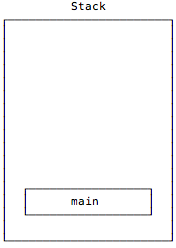# recursive

This is a page from my book, Functional Programming, Simplified

## Tail-Recursive Algorithms in Scala

“Tail recursion is its own reward.”

From the “Functional” cartoon on xkcd.com.

## Goals

The main goal of this lesson is to solve the problem shown in the previous lessons: Simple recursion creates a series of stack frames, and for algorithms that require deep levels of recursion, this creates a `StackOverflowError` (and crashes your program).

This is a page from my book, Functional Programming, Simplified

## A Visual Look at JVM Stacks and Frames

Given the background information of the previous lesson, let’s take a visual look at how the JVM stack and stack frames work by going back to our recursive sum function from the previous lesson.

Before the `sum` function is initially called, the only thing on the call stack is the application’s `main` method:Then main calls `sum` with `List(1,2,3)`, which I show here without the “List” to keep things simple:

This is a page from my book, Functional Programming, Simplified

## JVM Stacks and Stack Frames

For functions without deep levels of recursion, there’s nothing wrong with the algorithms shown in the previous lessons. I use this simple, basic form of recursion when I know that I’m working with limited data sets. But in applications where you don’t know how much data you might be processing, it’s important that your recursive algorithms are tail-recursive, otherwise you’ll get a nasty `StackOverflowError`.

For instance, if you run the `sum` function from the previous lessons with a larger list, like this:

This is a page from my book, Functional Programming, Simplified

## Recursion: Thinking Recursively

“To understand recursion,
one must first understand recursion.”

Stephen Hawking

## Goal

This lesson has one primary goal: to show that the thought process followed in writing the `sum` function follows a common recursive programming “pattern.” Indeed, when you write recursive functions you’ll generally follow the three-step process shown in this lesson.

Recursion: A Conversation Between Two Developers alvin May 29, 2017 - 11:07am

As an homage to one of my favorite Lisp books — an early version of what is now The Little Schemer — this lesson shows a little question and answer interaction that you can imagine happening between two Scala programmers.

Given this `sum` function:

``````def sum(list: List[Int]): Int = list match {
case Nil => 0
case x :: xs => x + sum(xs)
}``````

I hope this “conversation” will help drive home some of the points about how recursion works:

This is a page from my book, Functional Programming, Simplified

## Recursion: Visualizing the recursive `sum` Function

Another way to view recursion is with visual diagrams. To demonstrate this, I’ll use this rectangular symbol in this lesson to represent a function:## The first step

Using that symbol and a list with only three elements, the first `sum` function call looks like this:

This is a page from my book, Functional Programming, Simplified

## Recursion: How Recursive Scala Function Calls Work

An important point to understand about recursive function calls is that just as they “wind up” as they are called repeatedly, they “unwind” rapidly when the function’s end condition is reached.

In the case of the `sum` function, the end condition is reached when the `Nil` element in a `List` is reached. When `sum` gets to the `Nil` element, this pattern of the `match` expression is matched:

This is a page from my book, Functional Programming, Simplified

## Recursion: How to Write a ‘sum’ Function in Scala

With all of the images of the previous lesson firmly ingrained in your brain, let’s write a `sum` function using recursion!

## Sketching the `sum` function signature

Given a `List` of integers, such as this one:

``val list = List(1, 2, 3, 4)``

let’s start tackling the problem in the usual way, by thinking, “Write the function signature first.”